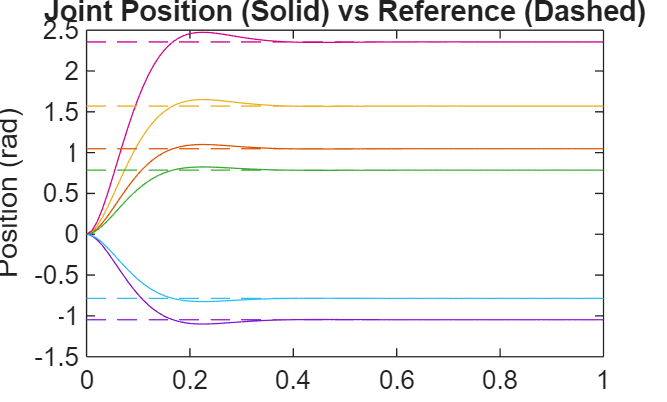# jointSpaceMotionModel

Model rigid body tree motion given joint-space inputs

## Description

The `jointSpaceMotionModel` object models the closed-loop joint-space motion of a manipulator robot, specified as a `rigidBodyTree` object. The motion model behavior is defined by the MotionType property.

For more details about the equations of motion, see Joint-Space Motion Model.

## Creation

### Syntax

``motionModel = jointSpaceMotionModel``
``motionModel = jointSpaceMotionModel("RigidBodyTree",tree)``
``motionModel = jointSpaceMotionModel(Name,Value)``

### Description

````motionModel = jointSpaceMotionModel` creates a motion model for a default two-joint manipulator.```

example

````motionModel = jointSpaceMotionModel("RigidBodyTree",tree)` creates a motion model for the specified `rigidBodyTree` object.```
````motionModel = jointSpaceMotionModel(Name,Value)` sets additional properties specified as name-value pairs. You can specify multiple properties in any order.```

## Properties

expand all

Rigid body tree robot model, specified as a `rigidBodyTree` object that defines the inertial and kinematic properties of the manipulator.

Natural frequency of error dynamics, specified as a scalar or n-element vector in Hz, where n is the number of nonfixed joints in the associated `rigidBodyTree` object in the RigidBodyTree property.

#### Dependencies

To use this property, set the MotionType property to `"ComputedTorqueControl"` or `"IndependentJointMotion"`.

Damping ratio of the second-order error dynamics, specified as a scalar or n-element vector of real values, where n is the number of nonfixed joints in the associated `rigidBodyTree` object in the RigidBodyTree property. If a scalar is specified, then `DampingRatio` becomes an n-element vector of value `s`, where `s` is the specified scalar.

#### Dependencies

To use this property, set the MotionType property to `"ComputedTorqueControl"` or `"IndependentJointMotion"`.

Proportional gain for proportional-derivative (PD) control, specified as a scalar or n-by-n matrix, where n is the number of nonfixed joints in the associated `rigidBodyTree` object in the RigidBodyTree property. You must set the MotionType property to `"PDControl"`. If a scalar is specified, then `Kp` becomes `s*eye(n)`, where `s` is the specified scalar.

#### Dependencies

To use this property, set the MotionType property to `"PDControl"`.

Derivative gain for PD control, specified as a scalar or n-by-n matrix, where n in the number of nonfixed joints in the `rigidBodyTree` object in the RigidBodyTree property. If a scalar is specified, then `Kp` becomes `s*eye(n)`, where `s` is the specified scalar.

#### Dependencies

To use this property, set the MotionType property to `"PDControl"`.

Type of motion, specified as a string scalar or character vector that defines the closed-loop joint-space behavior that the object models. Options are:

• `"ComputedTorqueControl"` — Compensates for full-body dynamics and assigns the error dynamics specified in the NaturalFrequency and DampingRatio properties.

• `"IndependentJointMotion"` — Models each joint as an independent second-order system using the error dynamics specified by the `NaturalFrequency` and `DampingRatio` properties.

• `"PDControl"` — Uses proportional-derivative control on the joints based on the specified Kp and Kd properties.

## Object Functions

 `derivative` Time derivative of manipulator model states `updateErrorDynamicsFromStep` Update values of `NaturalFrequency` and `DampingRatio` properties given desired step response

## Examples

collapse all

This example shows how to create and use a `jointSpaceMotionModel` object for a manipulator robot in joint-space.

Create the Robot

`robot = loadrobot("kinovaGen3","DataFormat","column","Gravity",[0 0 -9.81]);`

Set Up the Simulation

Set the timespan to be 1 s with a timestep size of 0.01 s. Set the initial state to be the robots, home configuration with a velocity of zero.

```tspan = 0:0.01:1; initialState = [homeConfiguration(robot); zeros(7,1)];```

Define the a reference state with a target position, zero velocity, and zero acceleration.

`targetState = [pi/4; pi/3; pi/2; -pi/3; pi/4; -pi/4; 3*pi/4; zeros(7,1); zeros(7,1)];`

Create the Motion Model

Model the system with computed torque control and error dynamics defined by a moderately fast step response with 5% overshoot.

```motionModel = jointSpaceMotionModel("RigidBodyTree",robot); updateErrorDynamicsFromStep(motionModel,.3,.05);```

Simulate the Robot

Use the derivative function of the model as the input to the `ode45` solver to simulate the behavior over 1 second.

`[t,robotState] = ode45(@(t,state)derivative(motionModel,state,targetState),tspan,initialState);`

Plot the Response

Plot the positions of all the joints actuating to their target state. Joints with a higher displacement between the starting position and the target position actuate to the target at a faster rate than those with a lower displacement. This leads to an overshoot, but all of the joints have the same settling time.

```figure plot(t,robotState(:,1:motionModel.NumJoints)); hold all; plot(t,targetState(1:motionModel.NumJoints)*ones(1,length(t)),"--"); title("Joint Position (Solid) vs Reference (Dashed)"); xlabel("Time (s)") ylabel("Position (rad)");```Craig, John J. Introduction to Robotics: Mechanics and Control. Upper Saddle River, NJ: Pearson Education, 2005.

 Spong, Mark W., Seth Hutchinson, and Mathukumalli Vidyasagar. Robot Modeling and Control. Hoboken, NJ: Wiley, 2006.